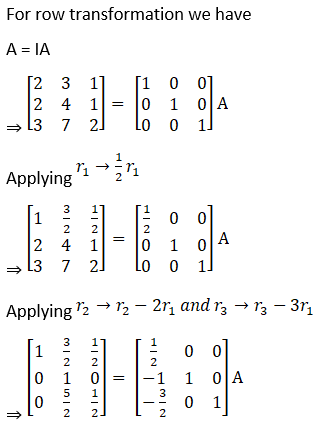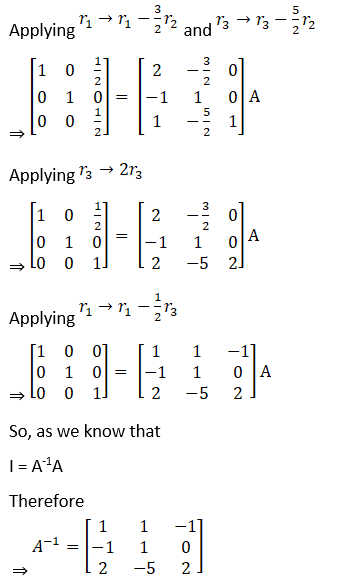# RD Sharma Solutions For Class 12 Maths Exercise 7.2 Chapter 7 Adjoint and Inverse of a Matrix

RD Sharma Solutions for Class 12 Maths Exercise 7.2 Chapter 7 Adjoint and Inverse of a Matrix are provided here. In this exercise, students will primarily get conceptual knowledge about the elementary transformation of a matrix. Class 12 is a major turning point for students to help them shape and achieve their future goals.

The RD Sharma Solutions for these problems are prepared by the experts at BYJU’S in a comprehensive manner, which improves the problem-solving capacity of students. The PDF of RD Sharma Solutions for Class 12 Maths Exercise 7.2 of Chapter 7 Adjoint and Inverse of a Matrix are provided here.

## RD Sharma Solutions For Class 12 Maths Chapter 7 Adjoint and Inverse of a Matrix Exercise 7.2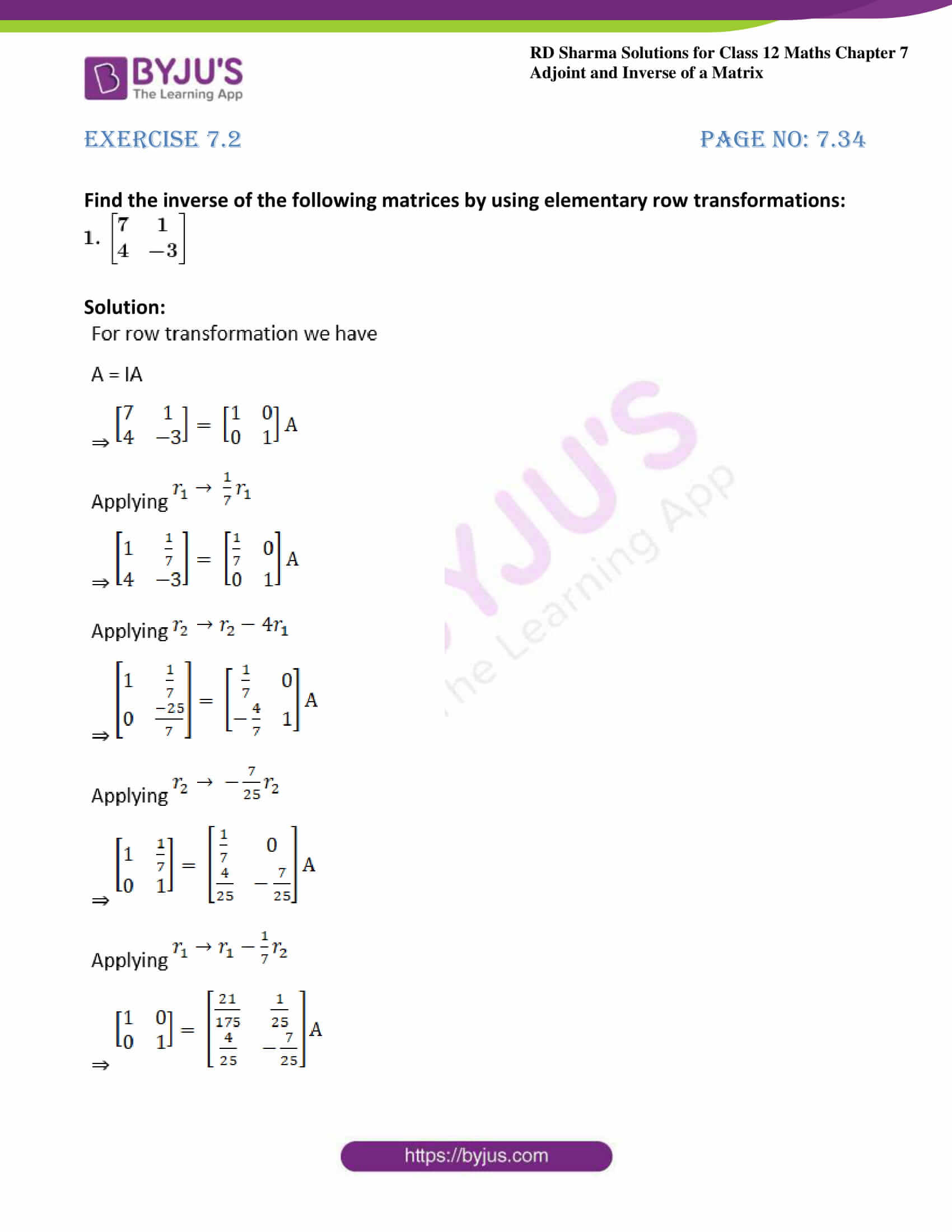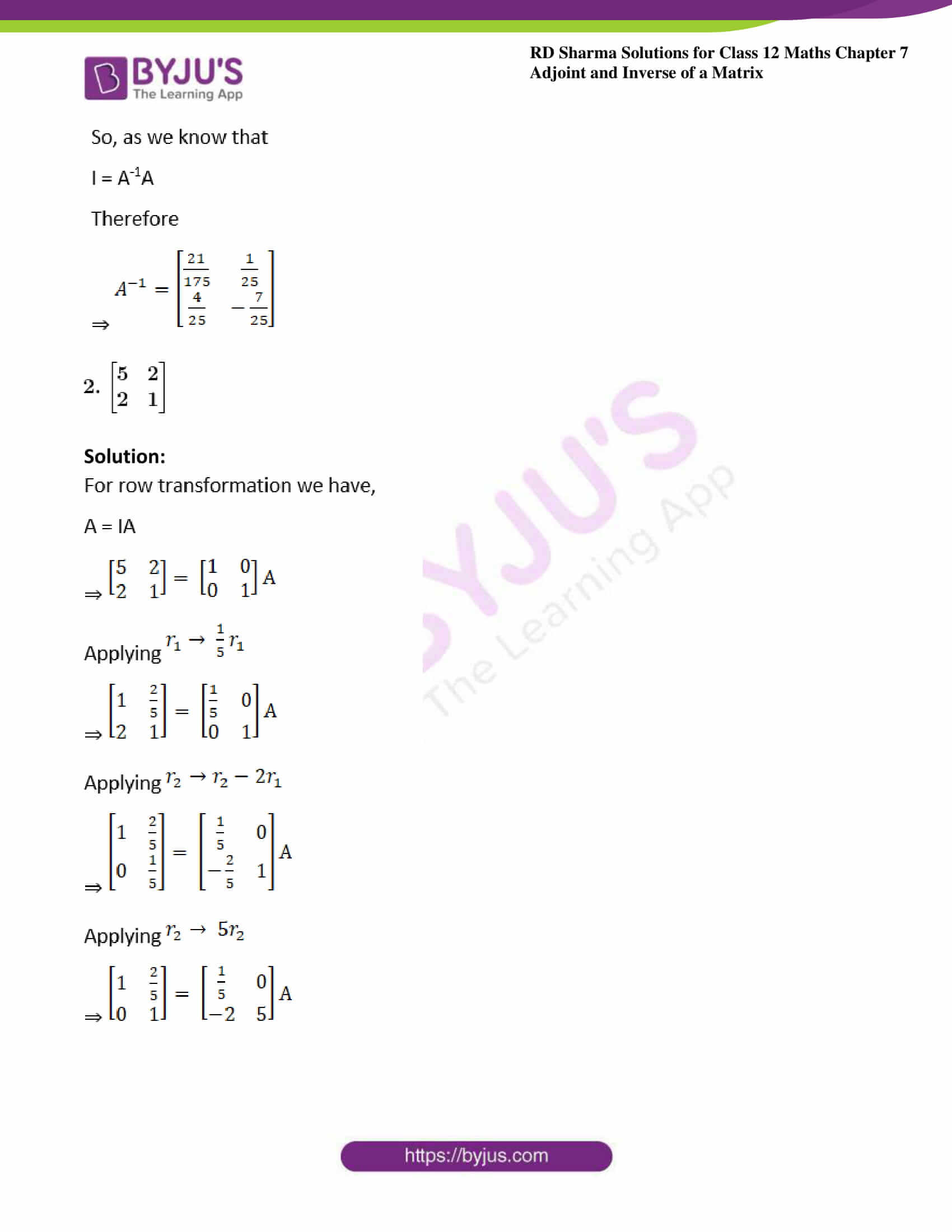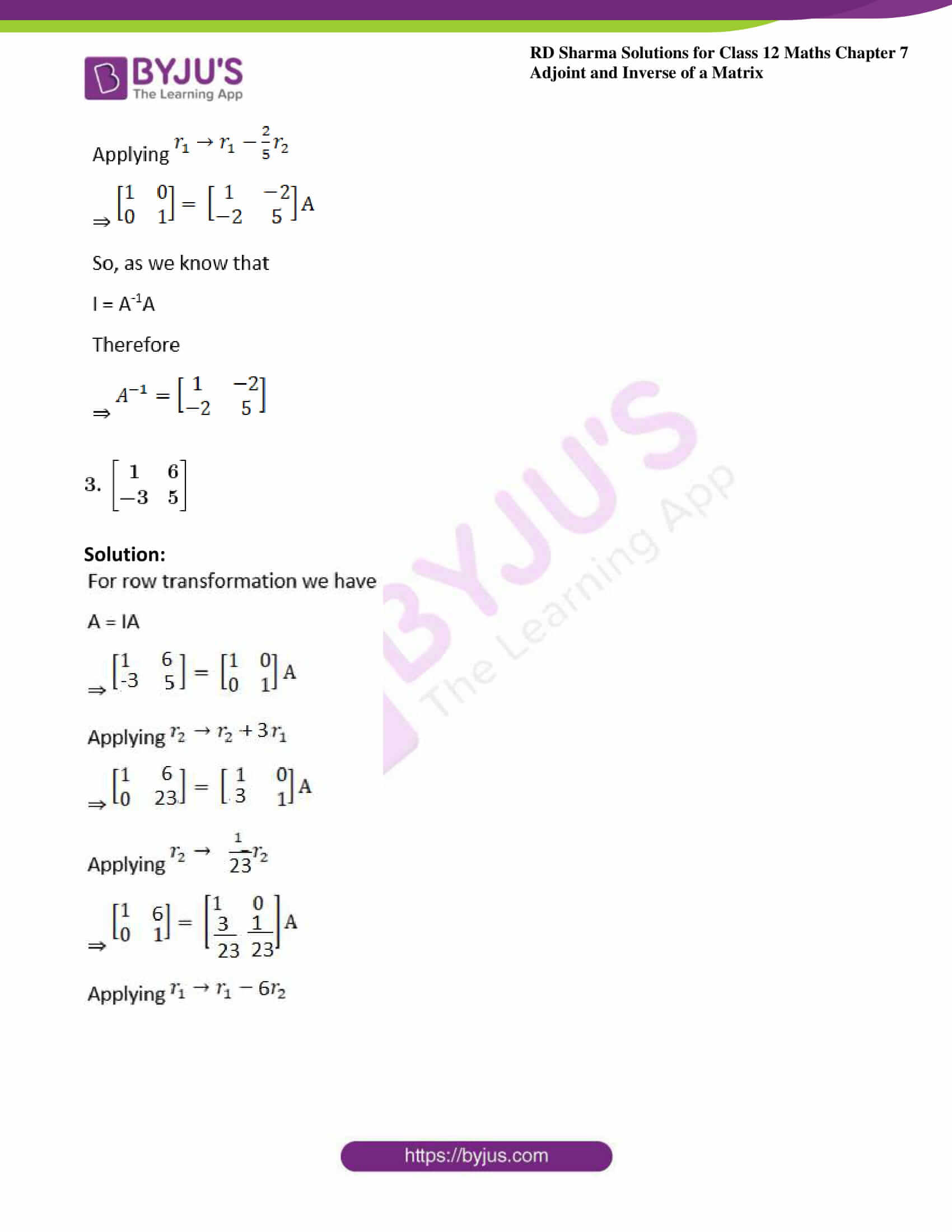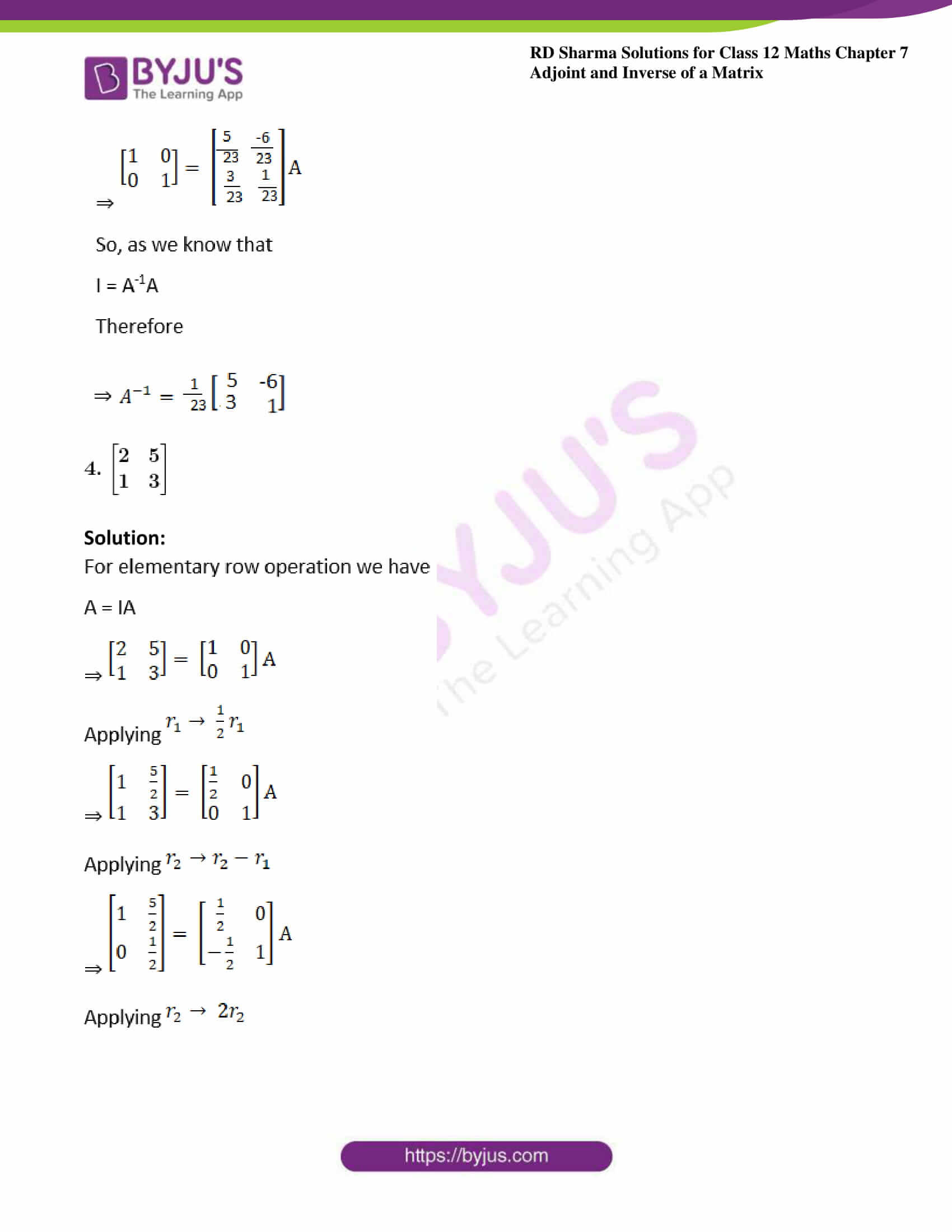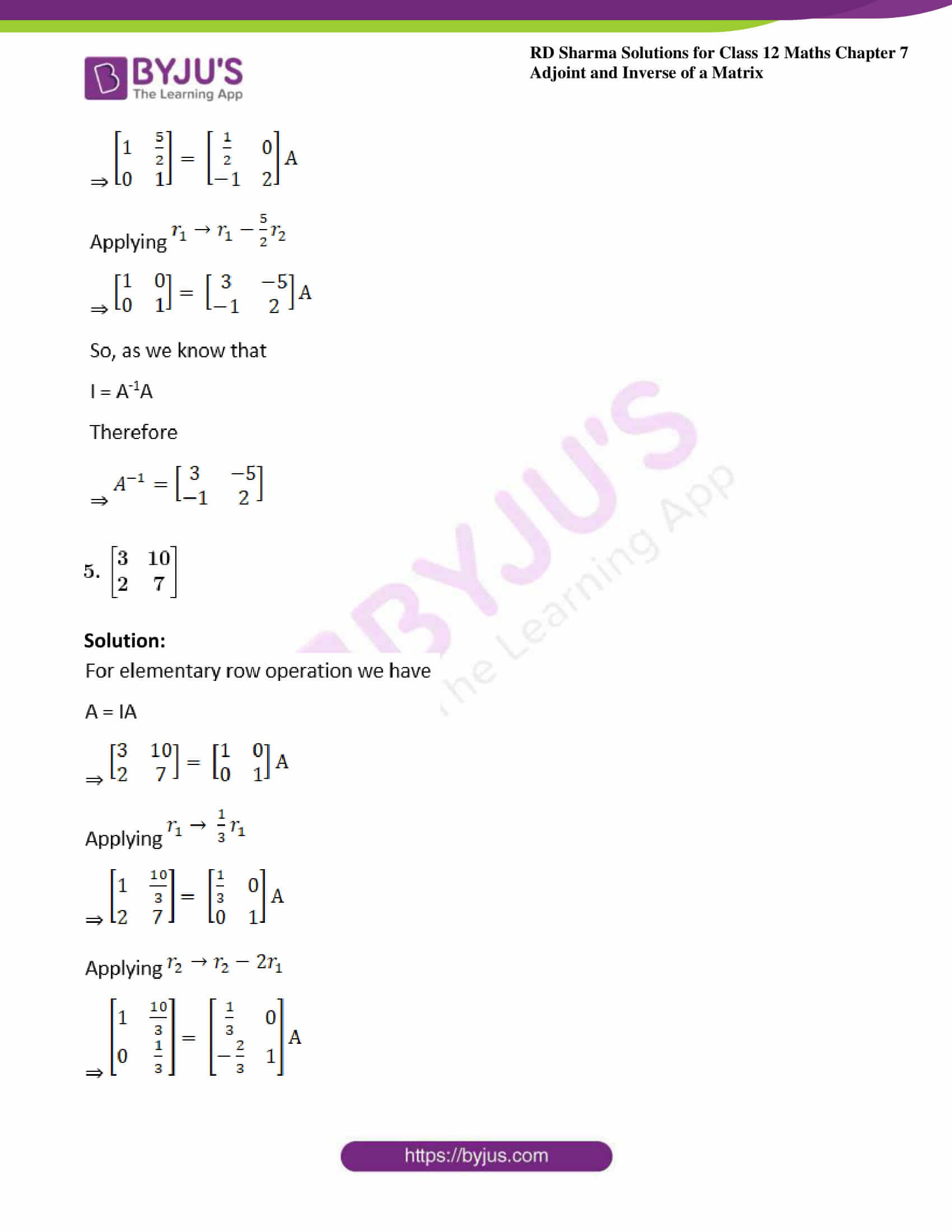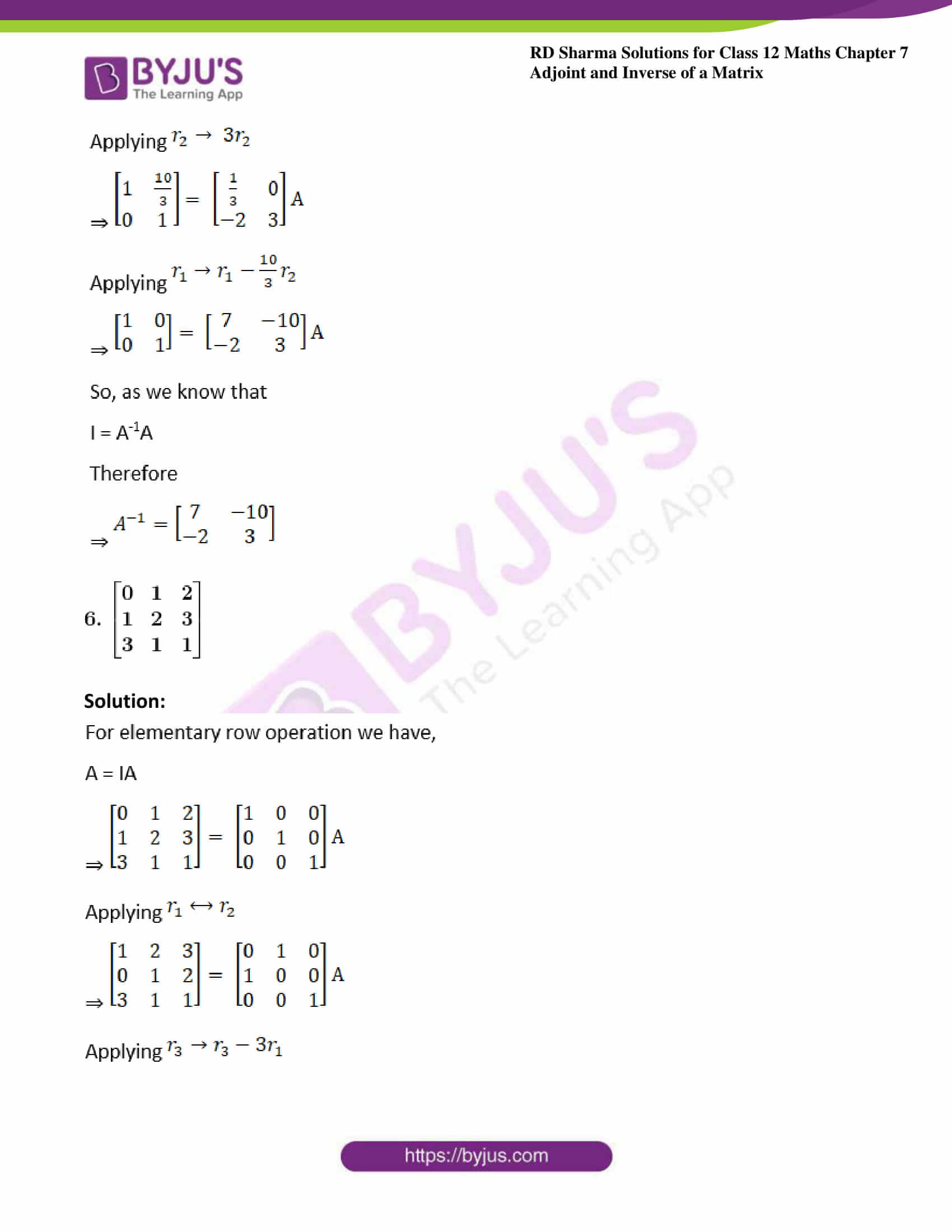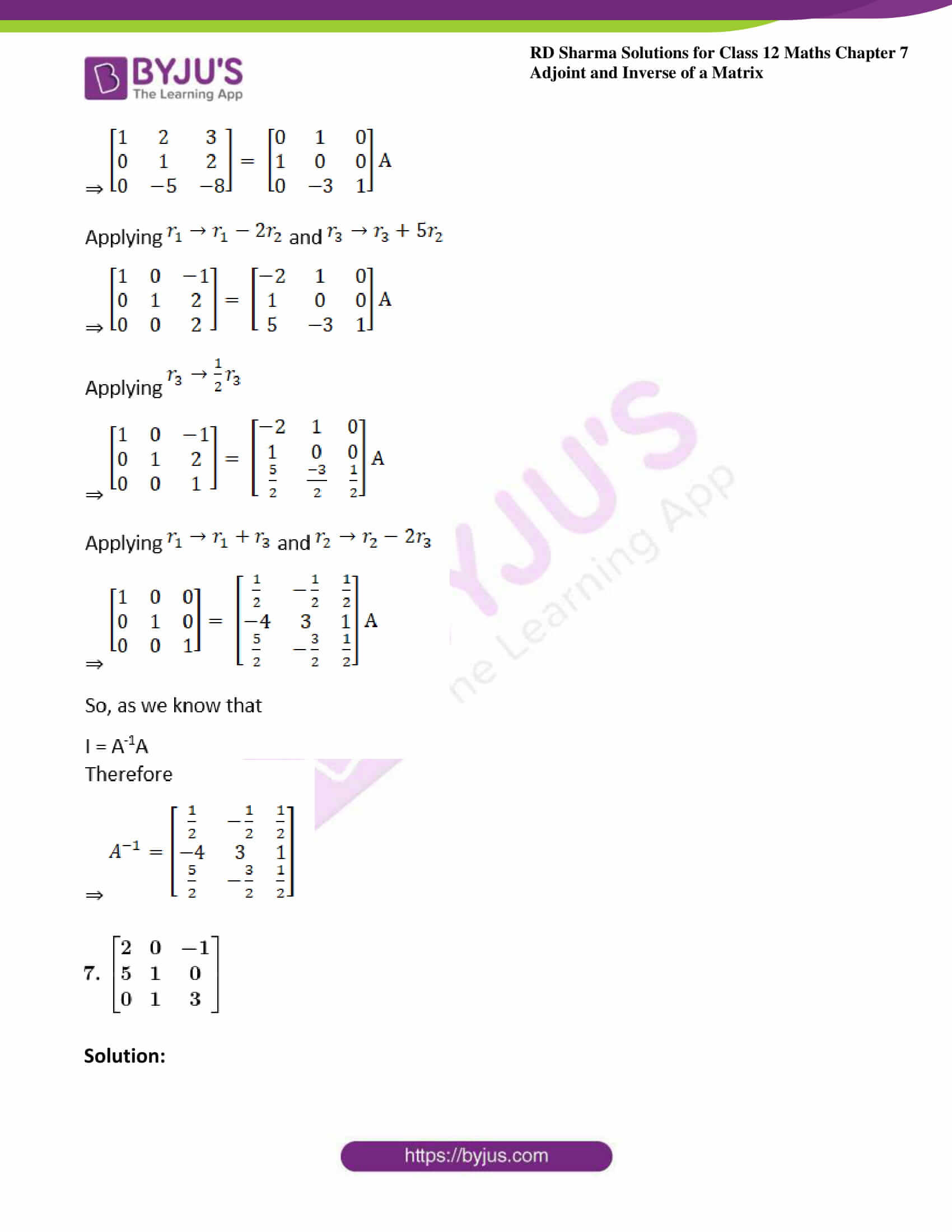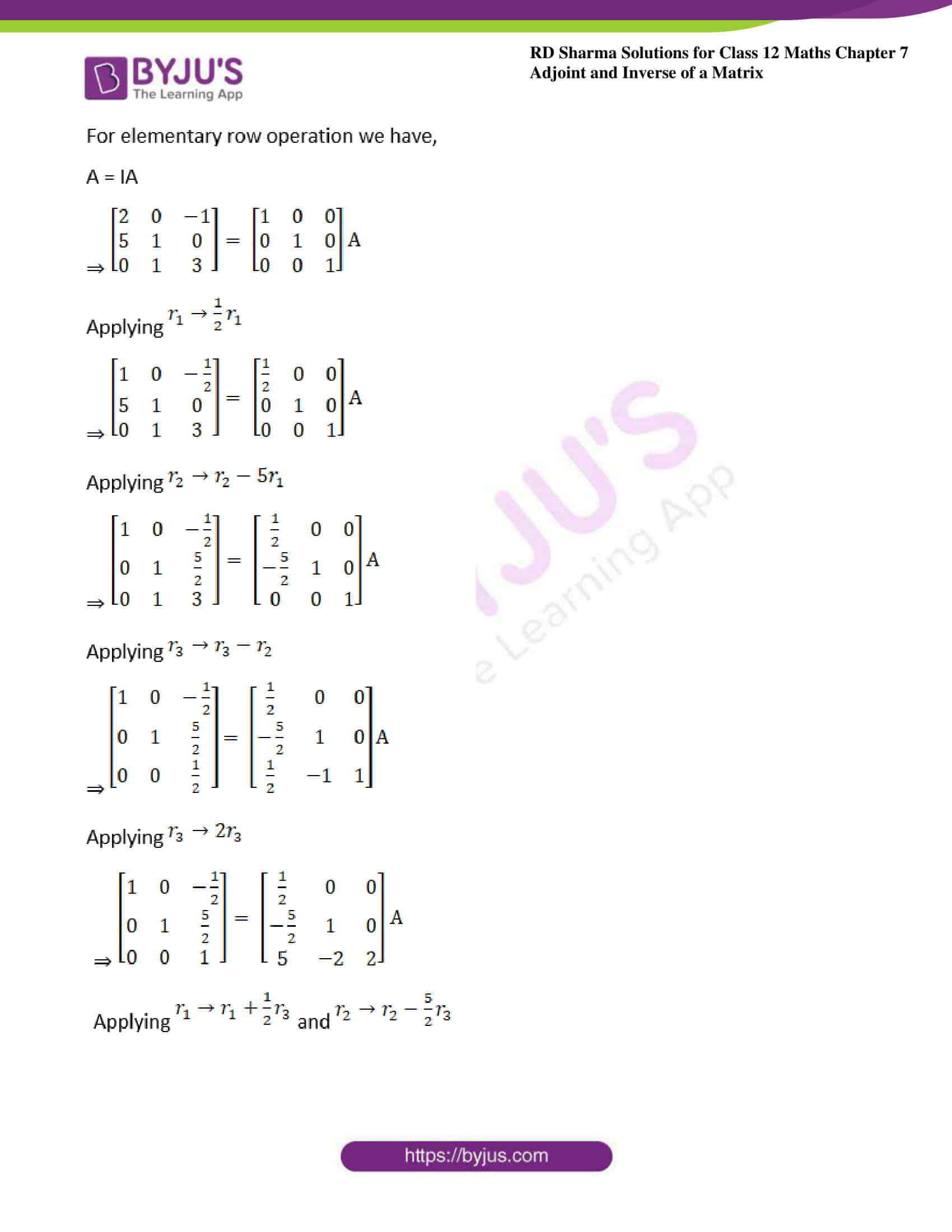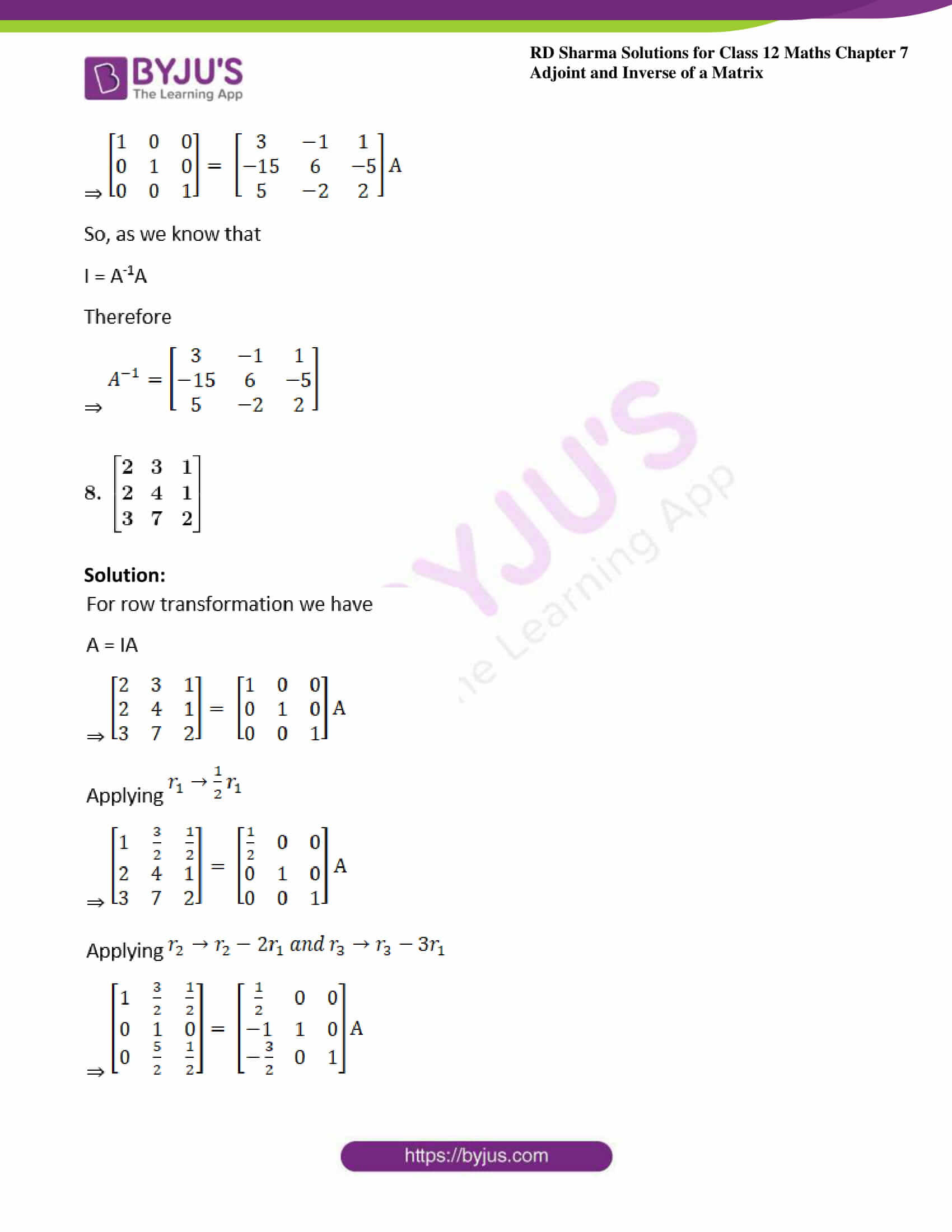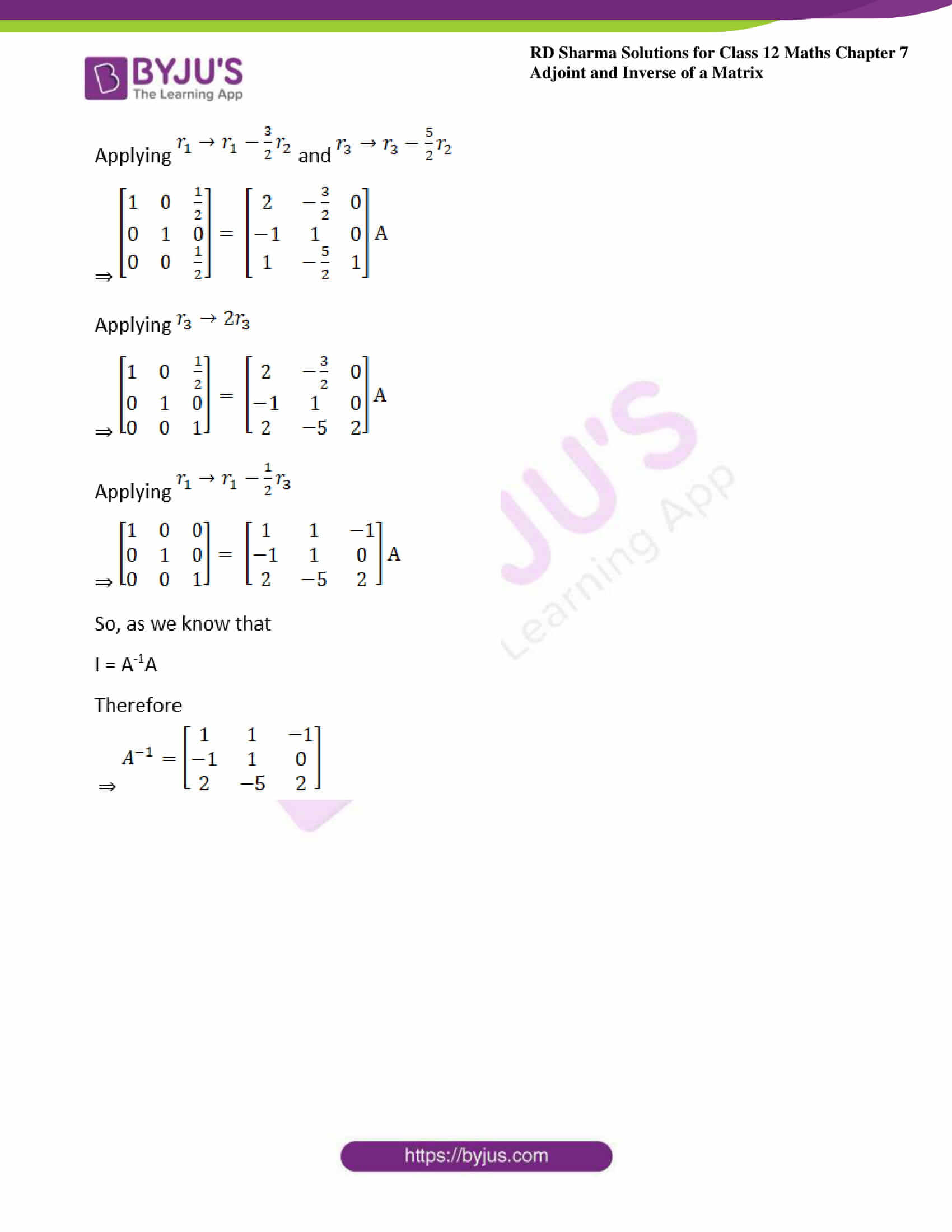### Access another exercise of RD Sharma Solutions For Class 12 Maths Chapter 7 – Adjoint and Inverse of a Matrix

Exercise 7.1 Solutions

### Exercise 7.2 Page No: 7.34

Find the inverse of the following matrices by using elementary row transformations: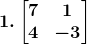Solution: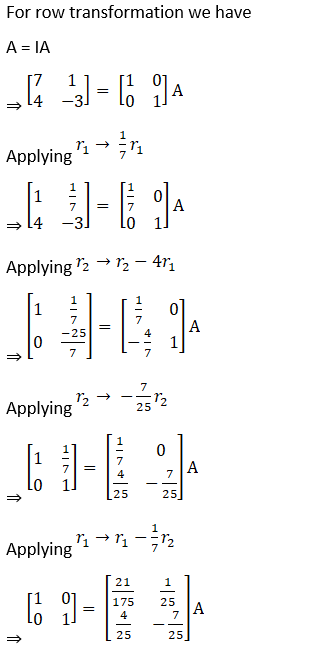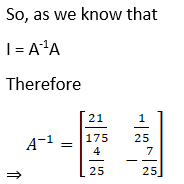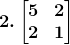Solution: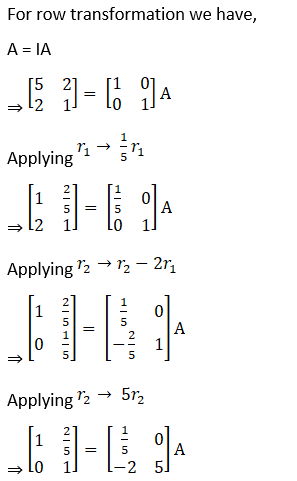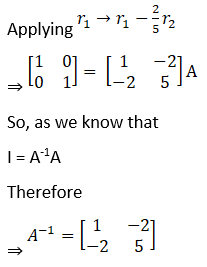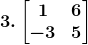Solution: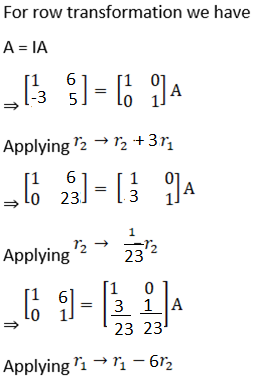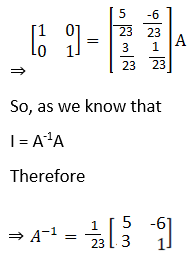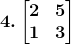Solution: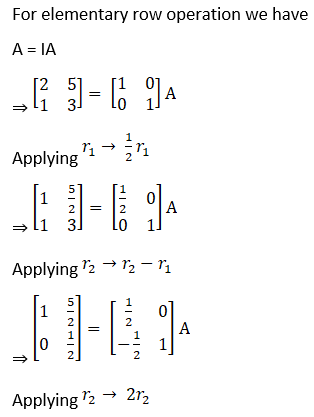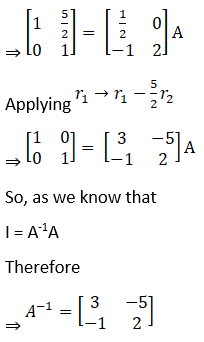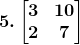Solution: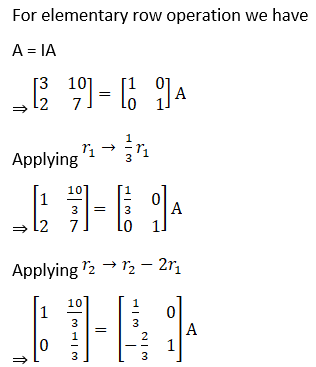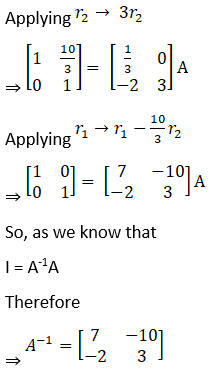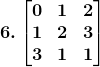Solution: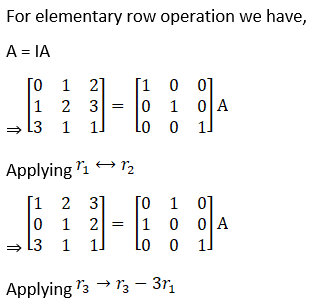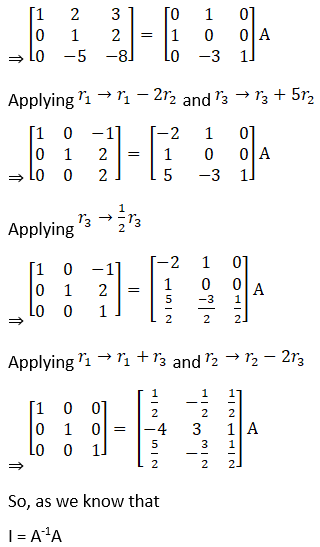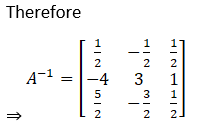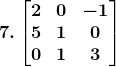Solution: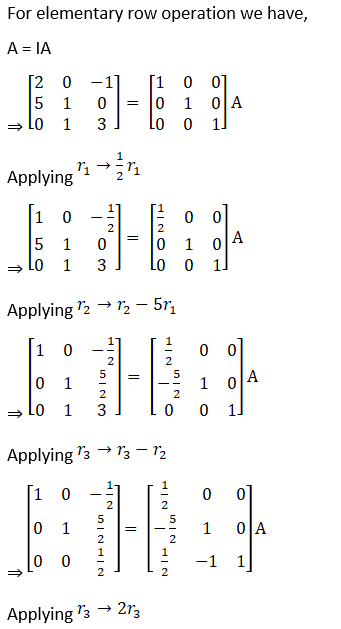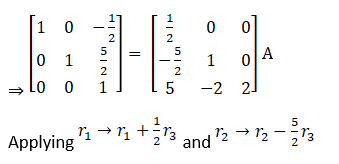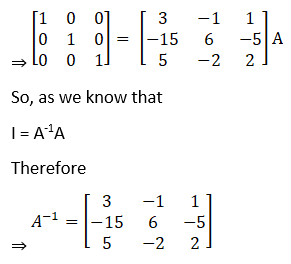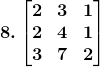Solution: